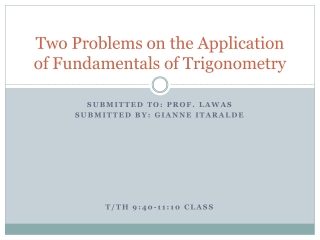DownloadDownload Presentationtrig project

# trig project

Download Presentation## trig project

- - - - - - - - - - - - - - - - - - - - - - - - - - - E N D - - - - - - - - - - - - - - - - - - - - - - - - - - -
##### Presentation Transcript

1. Two Problems on the Application of Fundamentals of Trigonometry Submitted to: Prof. Lawas Submitted by: Gianne Itaralde T/th 9:40-11:10 class

2. Problem #1 A boat with only one passenger is lost at sea. The passenger, being tired and all, is losing hope that he might not find land anymore and so, he slept. After a few hours, he woke up and saw a 115-feet high lighthouse from a distance. The angle of elevation from the boat to the top of the lighthouse is 10°. How far does he need to travel to get to land?

3. Illustration 115 feet 10 ° _?_ feet

4. Solution A 115 feet Angle: A = B = C = Line: a = b = 80 ° 652.1974 ft 10 ° 115 ft. c b 90 ° 10 ° B unknown C a To find Angle A: To find Line a: Tan A = _a_ b Equation (SOH-CAH-TOA) Tan A is equal to a divided by b Tan 80 = _a_ 115 Substitute 115 (Tan 80° = _a_) 115 115 Multiply both sides by 115 Tan A = Opposite Side / Adjacent Side Equation (SOH-CAH-TOA) Angle A = 180 – (Angle C + Angle B) Equation (Subtract the sum of Angles C and B from 180°) Angle A = 180 – (90 + 10) Substitute a = 652.1974 feet Answer 115 (5.6713) = a Solve for Tan 80° 652.1974 = a Multiply Angle A = 80 ° Answer

5. Answer to Problem #1 The passenger needs to travel 652.1974 feet to get to land. 652.1974 feet

6. Problem #2 The leaning tower of Pisa is tilted at an angle of 4.6° from the vertical. An observer standing 100m from the base of the tower measures the angle of elevation to be 30°. Find the height of the tower.

7. Illustration 4.6° _?_ m 100m 30°

8. Solution A Angle: A = B = C = Line: a = b = 64.6° 100m 30° 55.3526m 4.6° c 85.4° b 100m 30° B C a To find Angle C: To find Line b: To find Angle A: Sin A = Sin B a b Equation (Use the Law of Sines) Law of Sines: Sin A/a = Sin B/b = Sin C/c 0.9033 = 0.5 100 b Solve for Sin 64.6 and Sin 30 Sin 64.6 = Sin 30 100 b Substitute 100 ( 0.9033 = 0.5 ) 100 100 b Multiply both sides by 100 b (0.9033) = __50__ 0.9033 0.9033 Divide both sides by 0.9033 b ( 0.9033 = 50 ) b b Multiply both sides by b Angle A = 180 - (30 ° +85.4 °) Subtract the sum of Angles B and C from 180 Angle C = 85.4° Solve and that will be the answer b = 55.3526m Solve and that will be your answer Angle A = 64.6° Solve and that will be the answer Angle C = 90 – 4.6 Subtract 4.6 ° from 90 °

9. Answer to Problem #2 The Leaning Tower of Pisa is 55.3526m high. 55.3526m

10. End of Presentation Gianne Itaralde’s Project for funtrig Sources of images: http://static.ddmcdn.com http://www.dragoart.com http://data.greatbuildings.com http://bou.me